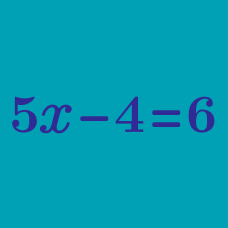Algebra

# Isolating a Variable

Solve the following for $Y$ in terms of $X:$ $X = 6Y .$

Given the equation $X = 2 Y + 7,$ solve for $Y$ in terms of $X$.

Solve the following for $Y$ in terms of $X:$ $\frac{Y+2}{X+7} = 1.$

Joshua has 5 times as many toys as Kevin.

Joshua has 8 more toys than Larry.

If Larry has $L$ toys and Kevin has $K$ toys, which of the following expresses $K$ in terms of $L$?

Given the equation $X = 3 Y + 6,$ solve for $Y$ in terms of $X$.

×